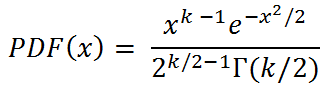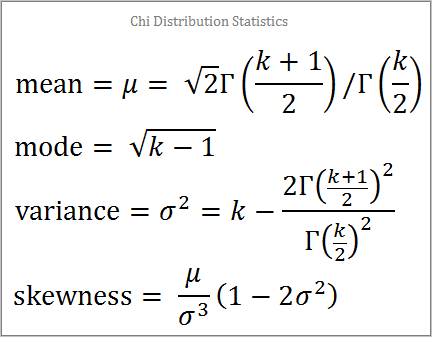# Chi Distribution Random Number Generator

The chi-distribution with k degrees of freedom, χ k , is the distribution of the square root of the sum of the squares of k standard normal random variables. For example, if R, S, and T are drawn from a normal distribution with a mean of 0 and variance of 1, then

sqrt(R² + S² + T²)

has a χ3 distribution. You can use the calculator below to generate random χ k variables.

 Degrees of Freedom =       N = Decimal Precision integer tenths hundredths thousandths Display comma separated vertical

The PDF of the chi distribution is given by the equationThe theoretical mean, median, mode, variance, and skewness of the chi distribution depend upon k. The equations are more complicated than for the chi-squared distribution.The median of the chi-distribution does not have a closed form, however, it can be closely approximated with the function below:

k = 1: median = 0.674
k = 2: median = 1.177
k = 3: median = 1.5838
k > 3: median = 0.886k0.5336